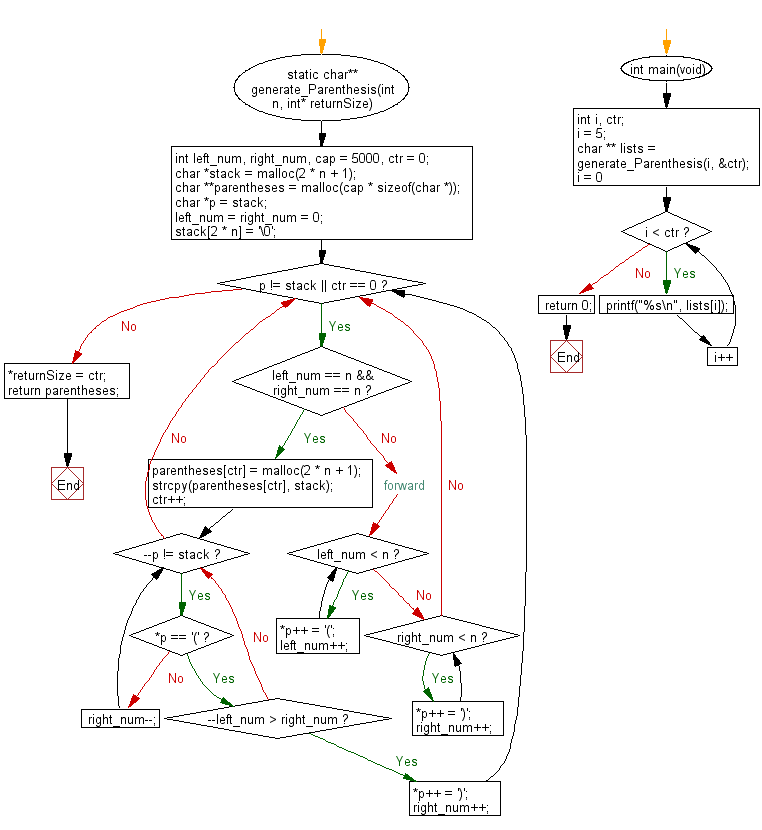﻿ C Program: Combinations of parentheses from n pairs# C Exercises: Generate all combinations of well-formed parentheses from n given pairs of parentheses

## C Programming Practice: Exercise-11 with Solution

Write a C programming to generate all combinations of well-formed parentheses from n given pairs of parentheses.

```Example:
Input  n = 5
Output:
(()())(())
(()())()()
(())((()))
(())(()())
(())(())()
(())()(())
(())()()()
()(((())))
()((()()))
()((())())
()((()))()
()(()(()))
()(()()())
()(()())()
()(())(())
()(())()()
()()((()))
()()(()())
()()(())()
()()()(())
()()()()()
```

C Code:

``````#include <stdio.h>
#include <stdlib.h>
#include <string.h>

static char** generate_Parenthesis(int n, int* returnSize)
{
int left_num, right_num, cap = 5000, ctr = 0;
char *stack = malloc(2 * n + 1);
char **parentheses = malloc(cap * sizeof(char *));

char *p = stack;
left_num = right_num = 0;
stack[2 * n] = '\0';

while (p != stack || ctr == 0) {
if (left_num == n && right_num == n) {
parentheses[ctr] = malloc(2 * n + 1);
strcpy(parentheses[ctr], stack);
ctr++;

while (--p != stack) {
if (*p == '(') {
if (--left_num > right_num) {
*p++ = ')';
right_num++;
break;
}
} else {
right_num--;
}
}
} else {
/* forward */
while (left_num < n) {
*p++ = '(';
left_num++;
}
while (right_num < n) {
*p++ = ')';
right_num++;
}
}
}

*returnSize = ctr;
return parentheses;
}

int main(void)
{
int i, ctr;
i = 5;
char ** lists = generate_Parenthesis(i, &ctr);
for (i = 0; i < ctr; i++) {
printf("%s\n", lists[i]);
}
return 0;
}
``````

Sample Output:

```((((()))))
(((()())))
(((())()))
(((()))())
(((())))()
((()(())))
((()()()))
((()())())
((()()))()
((())(()))
((())()())
((())())()
((()))(())
((()))()()
(()((())))
(()(()()))
(()(())())
(()(()))()
(()()(()))
(()()()())
(()()())()
(()())(())
(()())()()
(())((()))
(())(()())
(())(())()
(())()(())
(())()()()
()(((())))
()((()()))
()((())())
()((()))()
()(()(()))
()(()()())
()(()())()
()(())(())
()(())()()
()()((()))
()()(()())
()()(())()
()()()(())
()()()()()
```

Flowchart:## C Programming Code Editor:

What is the difficulty level of this exercise?

Test your Programming skills with w3resource's quiz.

﻿

## C Programming: Tips of the Day

What is the difference between printf() and puts() in C?

puts is simpler than printf but be aware that the former automatically appends a newline. If that's not what you want, you can fputs your string to stdout or use printf.

Ref :https://bit.ly/3f2fwcH